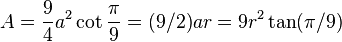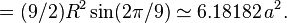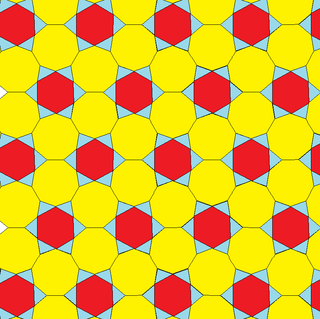# Nonagon facts for kids

Kids Encyclopedia Facts
(Redirected from Enneagon)

A nonagon or 9-gon is a shape with 9 sides and 9 corners.

## Regular nonagon

All sides of a regular nonagon are the same length. Each corner is 147.27°. All corners added together equal 6840°.

### Area

The amount of space a regular nonagon takes up is$A = \frac{9}{4}a^2\cot\frac{\pi}{9}=(9/2)ar = 9r^2\tan(\pi/9)$$= (9/2)R^2\sin(2\pi/9)\simeq6.18182\,a^2.$

a is the length of one of its sides.

## Tilings

The regular enneagon can tessellate the euclidean tiling with gaps. These gaps can be filled with regular hexagons and isosceles triangles. In the notation of symmetrohedron this tiling is called H(*;3;*;) with H representing *632 hexagonal symmetry in the plane.## Images for kidsNonagon Facts for Kids. Kiddle Encyclopedia.